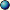### Predefined Parameters : Escapetime

Fractal type Escapetime offers several predefined parameters, partially depending on the formula type. These parameters provide a way to access some seettings outside a formula context, for example the rotation angle or the screen resolution.

Please note: Different fractal types (currently Escapetime and Quaternion) offer different predefined parameters.

The following parameters exist:Transformation Iteration Coloringalpha real r/w real r/w real r/wangle real r/o real r/o real r/ocenter complex r/o complex r/o complex r/ocorner_lefttop complex r/o complex r/o complex r/ocorner_rightbottom complex r/o complex r/o complex r/oe real r/o real r/o real r/oheight real r/o real r/o real r/oindex - – - - – - real rwlastsqr - – - real r/o - – -magn real r/o real r/o real r/omaxiter real r/o real r/o real r/onumiter real r/w real r/w real r/wpi real r/o real r/o real r/opixel complex r/w complex r/w complex r/wpixelcolor - – - - – - color r/wrandom complex r/o complex r/o complex r/orandomrange int r/o int r/o int r/orotation_center complex r/o complex r/o complex r/oscreenmax complex r/o complex r/o complex r/oscreenpixel complex r/o complex r/o complex r/osolid bool w/o bool w/o bool w/owhitesq real r/o real r/o creal r/owidth real r/o real r/o real r/ox real r/o real r/o real r/oy real r/o real r/o real r/oz complex r/w complex r/w complex r/wNotes:

The table lists the datatype of the predefined parameter and one of the following abbreviations:

• - – -: not available
• r/o means: read-only
• r/w means: read-write
• w/o means: write-only